Chapter 5, Problem 5.79EP### Organic And Biological Chemistry

7th Edition
STOKER + 1 other
ISBN: 9781305081079

#### Solutions

Chapter
Section### Organic And Biological Chemistry

7th Edition
STOKER + 1 other
ISBN: 9781305081079
Textbook Problem

# Which of the following structures represent esters?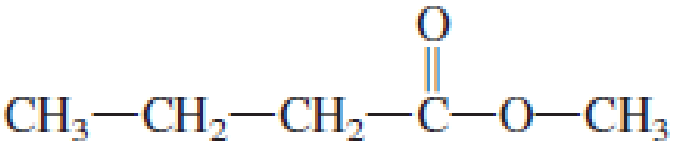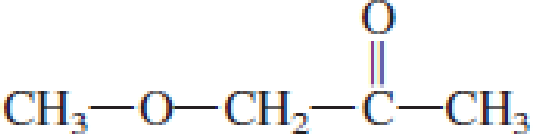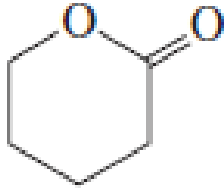Interpretation Introduction

Interpretation:

Among the given structures, the structures that represent esters have to be chosen.

Concept Introduction:

Carbonyl groups are the one which contain a double bond between carbon and oxygen atom.  Aldehydes and ketones possess this carbonyl functional group in it.  The structural representation of a carbonyl group can be given as shown below,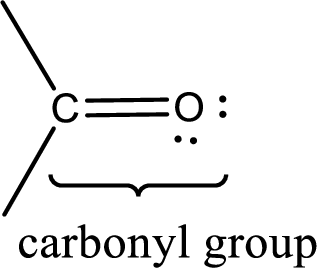If a hydroxyl group is attached to a carbonyl group means it is known as carboxyl group.  This can be represented as shown below,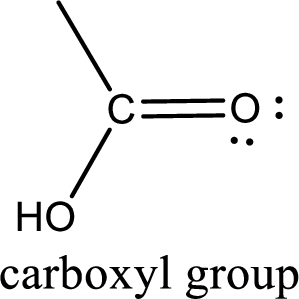Carboxylic acid derivatives are the ones that are synthesized from or converted to a carboxylic acid.  The generalized structural representation of carboxylic acid derivatives is shown below,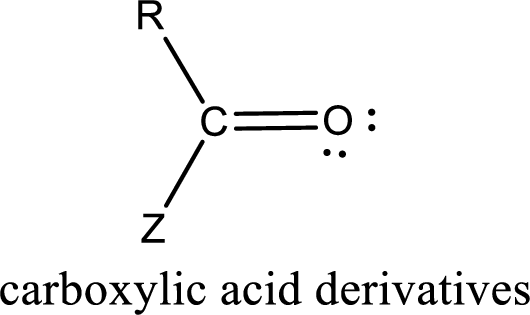The general structure of ester can be given as shown below,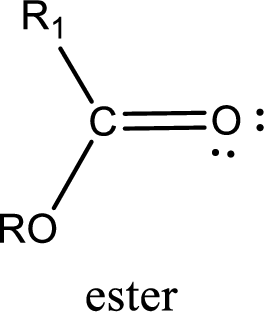The functional group of ester compound can be shown as given below,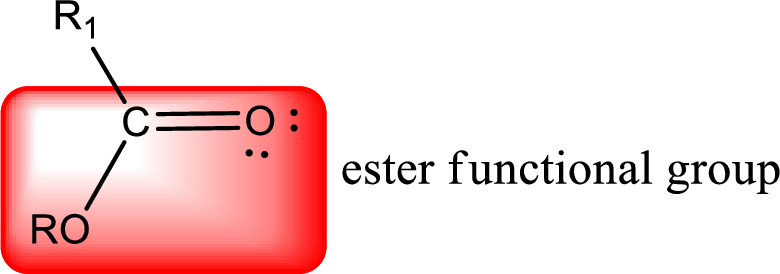Explanation

Reason for correct options:

The general structure of ester can be given as shown below,

Structure given in option (a) is,

Ester functional group is present in the above structure and it can be shown as,

Hence, this structure represents an ester.

Structure given in option (b) is,

Ester functional group is present in the above structure and it can be shown as,

Hence, this structure represents an ester...

### Still sussing out bartleby?

Check out a sample textbook solution.

See a sample solution

#### The Solution to Your Study Problems

Bartleby provides explanations to thousands of textbook problems written by our experts, many with advanced degrees!

Get Started